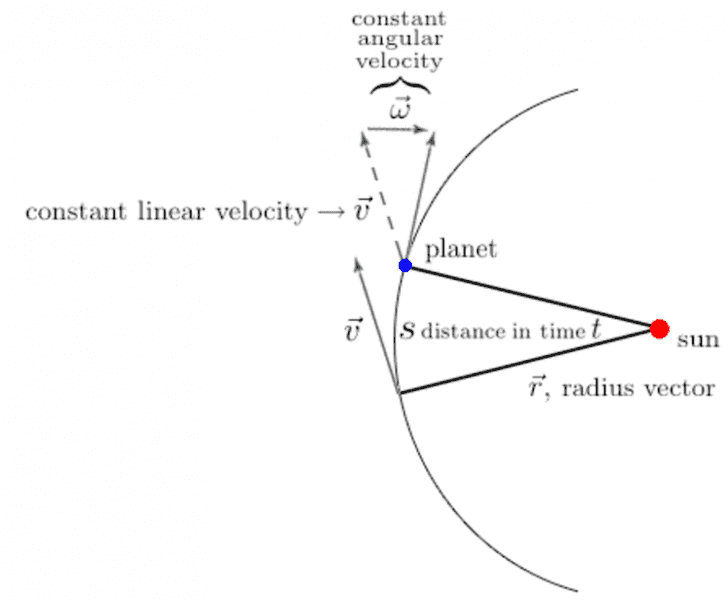# Deriving Newtonian law of gravitation

• TimeRip496
I agree that the OP's source is incorrect in several other ways. Better to stick to reliable texts or online sites.f

## Homework Statement## Homework Equations

$$\frac{\omega}{v}=\frac{s}{r}$$

## The Attempt at a Solution

How to get the equation above? Cause
$$\frac{s}{r}=\omega$$ & $$\frac{\omega}{v}=\frac{1}{r}$$ since v=rw.

Source: http://www.relativitycalculator.com/Newton_Universal_Gravity_Law.shtml

## Homework Statement

2. Homework Equations [/B]
$$\frac{\omega}{v}=\frac{s}{r}$$
This is not a correct equation. You can see that from the dimensions. LS = 1/distance and RS = distance / distance. Your source is not very well written and takes incomprehensible mathematical steps that appear to me to be wrong.

AM

This is not a correct equation. You can see that from the dimensions. LS = 1/distance and RS = distance / distance. Your source is not very well written and takes incomprehensible mathematical steps that appear to me to be wrong.

AM
Thanks! That's what I thought so. But without that step, I cannot continue and the subsequent steps that the source provides are useless in helping me to derive the Newtonian law of gravitation. Is there a correct way to derive the Newtonian laws of gravitation?

The author is trying to show how Kepler's laws lead to the ##F \propto 1/r^2## relationship for circular orbits.

I am not sure what the author of your source is trying to show with Kepler's second law. You can find the derivation of centripetal force in any first year physics textbook: ##a_c = v^2/r = \omega^2 r##.

From Kepler's third law, ##r^3 \propto T^2 \text{ or } \frac{r^3}{T^2} = \text{ constant}##. Since ##\omega = 2\pi/T## this means that ##r^3\omega^2 = K## or ##\omega^2 = \frac{K}{r^3}##

The gravitational force provides the centripetal force required for circular orbit, so ##g=v^2/r = \omega^2 r##, this means that ##g = Kr/r^3 = K/r^2##

AM

•TimeRip496
The angular velocity omega is r x v. The angular momentum vector is not even in the plane of the orbit. The angular momentum is normal to the plane of orbit. This source is clearly incorrect.

Sorry for the last post I was thinking of (specific) angular momentum not the angular velocity. I still think the source is unclear. A better explanation can be found in many textbooks.

Sorry for the last post I was thinking of (specific) angular momentum not the angular velocity. I still think the source is unclear. A better explanation can be found in many textbooks.
Your first post was correct. The direction of ##\vec{\omega}## is in the same direction as the angular momentum vector: perpendicular to the plane of rotation:

(1) ##\vec{L} = I\vec{\omega}##

and for a point mass
(2) ##\vec{L} = \vec{r} \times \vec{p}##

so:
(3) ##\vec{\omega} = \frac{\vec{L}}{I} = \frac{\vec{r} \times \vec{p}}{I}##

Since, for a point mass: ##I = m|\vec{r}|^2##, for a point mass (3) can be rewritten:
##\vec{\omega} =\frac{\vec{r} \times \vec{p}}{m|\vec{r}|^2} = \frac{\vec{r} \times \vec{v}}{|\vec{r}|^2}##

I agree that the OP's source is incorrect in several other ways. Better to stick to reliable texts or online sites.

AM